Open in App
Not now

# Python | Pandas dataframe.slice_shift()

• Last Updated : 23 Nov, 2018

Python is a great language for doing data analysis, primarily because of the fantastic ecosystem of data-centric python packages. Pandas is one of those packages and makes importing and analyzing data much easier.

Pandas` dataframe.slice_shift()` function is Equivalent to shift without copying data. The shifted data will not include the dropped periods and the shifted axis will be smaller than the original. This function simply drops the specified number of periods over the given axis in a specified direction.

Syntax: DataFrame.slice_shift(periods=1, axis=0)

Parameters :
periods : Number of periods to move, can be positive or negative

Return : shifted : same type as caller

Example #1: Use `slice_shift()` function to shift the index axis by 2 periods in a time-series data

 `# importing pandas as pd ` `import` `pandas as pd ` `  `  `# Creating row index values for dataframe ` `# We have taken time frequency to be of 12 hours interval ` `# Generating five index value using "period = 5" parameter ` `ind ``=` `pd.date_range(``'01/01/2000'``, periods ``=` `5``, freq ``=``'12H'``) ` `  `  `# Creating a dataframe with 4 columns ` `# using "ind" as the index for our dataframe ` `df ``=` `pd.DataFrame({``"A"``:[``1``, ``2``, ``3``, ``4``, ``5``],  ` `                   ``"B"``:[``10``, ``20``, ``30``, ``40``, ``50``],  ` `                   ``"C"``:[``11``, ``22``, ``33``, ``44``, ``55``], ` `                   ``"D"``:[``12``, ``24``, ``51``, ``36``, ``2``]}, index ``=` `ind) ` ` `  `# Print the dataframe ` `df `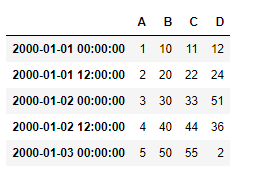Let’s use the `dataframe.slice_shift()` function to shift the index axis by 2 periods in positive direction

 `# shift index axis by two ` `# periods in positive direction ` `# axis = 0 is set by default ` `df.slice_shift(``2``, axis ``=` `0``) `

Output :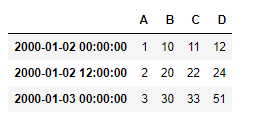Notice the index labels, first two labels are dropped but the data has been shifted by two periods in the positive direction.

We can also shift the index axis in negative direction by some periods

 `# shift index axis by two  ` `# periods in negative direction ` `# axis = 0 is set by default ` `df.slice_shift(``-``2``, axis ``=` `0``) `

Output :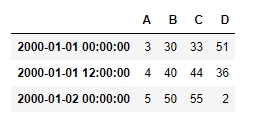Notice in the output, the data points has been shifted in the negative direction (i.e. upward) by 2 periods and the last two index labels has been removed.

Example #2: Use `slice_shift()` function to shift the column axis by 2 periods in a time-series data

 `# importing pandas as pd ` `import` `pandas as pd ` `  `  `# Creating row index values for our data frame ` `# Taken time frequency to be of 12 hours interval ` `# Generating five index value using "period = 5" parameter ` `  `  `ind ``=` `pd.date_range(``'01/01/2000'``, periods ``=` `5``, freq ``=``'12H'``) ` `  `  `# Creating a dataframe with 4 columns ` `# using "ind" as the index for our dataframe ` `df ``=` `pd.DataFrame({``"A"``:[``1``, ``2``, ``3``, ``4``, ``5``], ` `                   ``"B"``:[``10``, ``20``, ``30``, ``40``, ``50``], ` `                   ``"C"``:[``11``, ``22``, ``33``, ``44``, ``55``], ` `                   ``"D"``:[``12``, ``24``, ``51``, ``36``, ``2``]}, index ``=` `ind) ` ` `  `# shift column axis by two periods in positive direction ` `df.slice_shift(``2``, axis ``=` `1``) `

Output :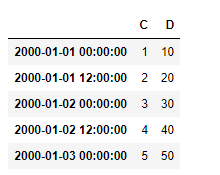In the output, we can see the first two column labels are removed and the data point along the column axis has been shifted by 2 periods in the positive direction.

We can also shift the column axis in negative direction by some periods

 `# shift column axis by two periods in negative direction ` `df.slice_shift(``-``2``, axis ``=` `0``) `

Output :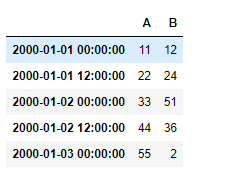In the output, we can see the last two column labels are removed and the data point along the column axis has been shifted by 2 periods in the negative direction (i.e. left).

My Personal Notes arrow_drop_up
Related Articles